Math resources Geometry Lines

Perpendicular lines

# Perpendicular lines

Here you will learn about perpendicular lines, including what they are and how to identify them.

Students will first learn about perpendicular lines as part of geometry in 4th grade.

## What are perpendicular lines?

Perpendicular lines are lines that intersect to form 90^{\circ} angles (right angles).

For example,

Perpendicular lines are seen in many common 2D shapes.

For example,

Each side of a square is perpendicular to the adjacent sides (the sides that touch).

This is why the corners of a square always form right angles.

There are also many examples of perpendicular lines in real life.

For example,

A table top and its legs are perpendicular to each other. Where the table top and the legs meet, a 90^{\circ} angle is formed.

### What are perpendicular lines?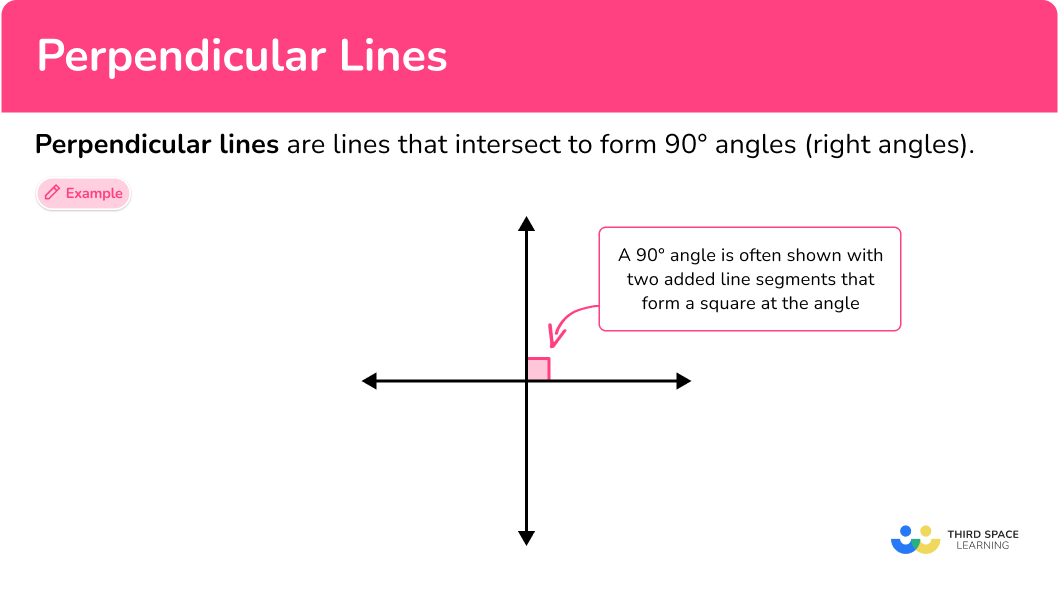## Common Core State Standards

How does this relate to 4th grade math?

• 4th Grade – Geometry (4.G.A.1)
Draw points, lines, line segments, rays, angles (right, acute, obtuse), and perpendicular and parallel lines. Identify these in two-dimensional figures

## How to identify perpendicular lines

In order to identify perpendicular lines:

1. Look for two lines that intersect.
2. Decide if the angle formed by the lines is a right angle.

## Perpendicular lines examples

### Example 1: two lines crossing

1. Look for two lines that intersect.

The image shows two lines that intersect (cross).

2Decide if the angle formed by the lines is a right angle.

There are acute angles and obtuse angles formed by the intersecting lines, but no right angles, so these lines are NOT perpendicular.

### Example 2: geometric lines

Are line AB and line CD perpendicular?

Look for two lines that intersect.

Decide if the angle formed by the lines is a right angle.

### Example 3: perpendicular lines in a rectangle

Highlight any sets of perpendicular lines in the rectangle.

Look for two lines that intersect.

Decide if the angle formed by the lines is a right angle.

### Example 4: lines in a triangle

Highlight any sets of perpendicular lines in the triangle.

Look for two lines that intersect.

Decide if the angle formed by the lines is a right angle.

### Example 5: perpendicular lines in the real world

Find a set of perpendicular lines.

Look for two lines that intersect.

Decide if the angle formed by the lines is a right angle.

### Example 6: perpendicular lines in real life

Find a set of perpendicular lines.

Look for two lines that intersect.

Decide if the angle formed by the lines is a right angle.

### Teaching tips for perpendicular lines

• Worksheets that show a variety of 2D shapes or real world pictures can be useful for this skill, along with everyday 2D and 3D objects found in the classroom. Encourage students to look for and identify perpendicular lines in a variety of places.

• Provide practice problems that go beyond asking students to just identify them but also ask them to create and explain perpendicular lines in a variety of scenarios. This shows students’ knowledge of the topic on multiple levels.

• Encourage students to measure different angles formed by two intersecting lines with a protractor and use the measurement to explain whether or not the lines are perpendicular.

### Easy mistakes to make

• Confusing parallel and perpendicular lines
Since they are often introduced together, in the beginning it is easy for students to confuse these two vocabulary words. With enough practice and real world application, students will learn the difference.

• Mixing up a third or fourth line
When more than two lines are shown, it can be hard to consider the relationship of only two lines at a time. Encourage students to highlight where two lines intersect or to physically cover the other lines, when deciding if two lines are perpendicular or not.
For example,

Students can…
Highlight two lines: Cover the extra lines:

### Practice perpendicular lines questions

1. Which of the given lines look perpendicular?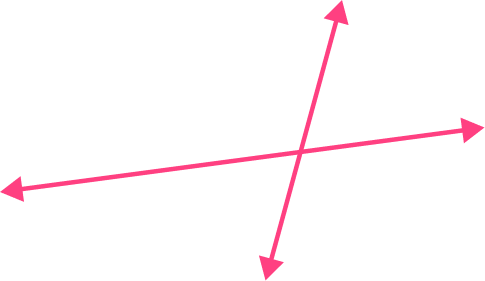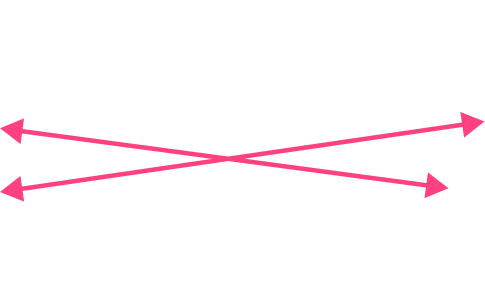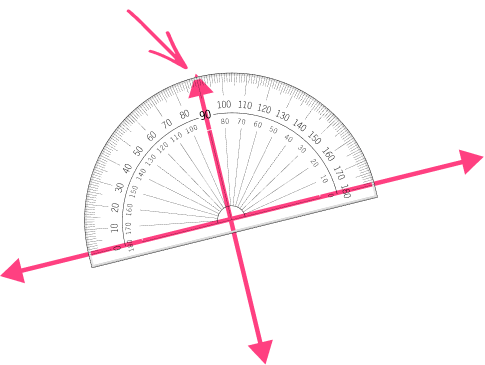These two lines look like they form right angles where they intersect.

Measuring the angle with the protractor proves that they do, and so these lines are perpendicular.

2. Which of the given lines look perpendicular to each other?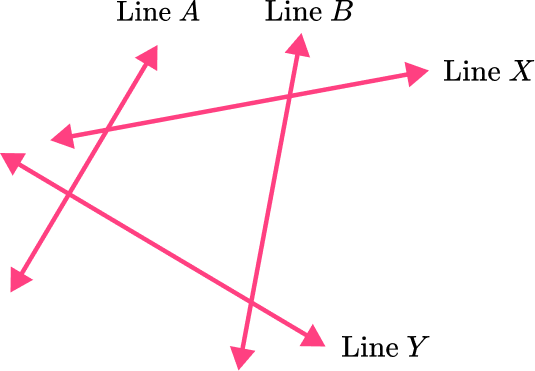line A and line Yline B and line Xline A and line Bline X and line Y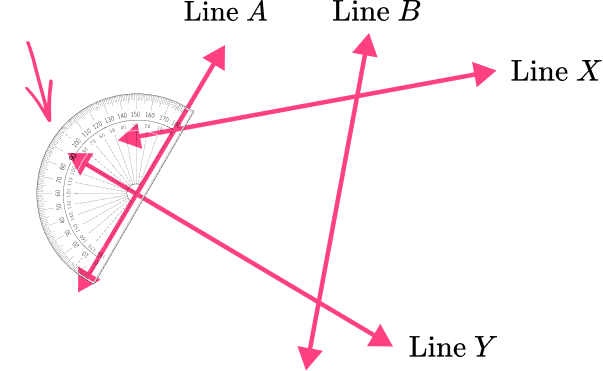The angles formed by line A crossing line Y look like right angles.

Measuring the angle with the protractor proves that they are, and so these lines are perpendicular.

3. Which shape has perpendicular lines?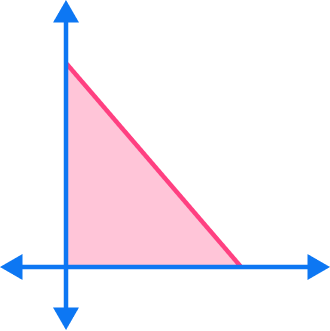A right triangle is formed when the base and the height are perpendicular to each other – creating a right angle (90^{\circ}).

4. Which shape DOES NOT have perpendicular lines?The lines that make up this trapezoid form acute and obtuse angles, but no right angles (90^{\circ}).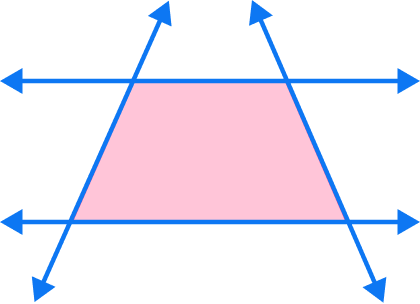This trapezoid DOES NOT have perpendicular lines.

5. Which lines on the ice cream look perpendicular?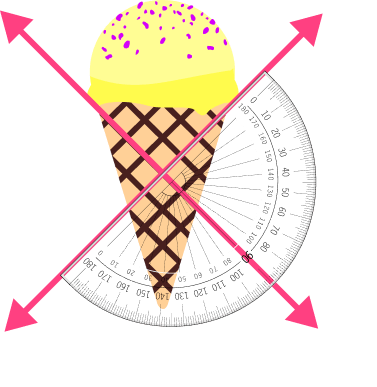Measuring with the protractor proves that there are four right angles (90^{\circ}) formed by the crossing lines, so these lines are perpendicular.

6. Which lines on the barn look perpendicular?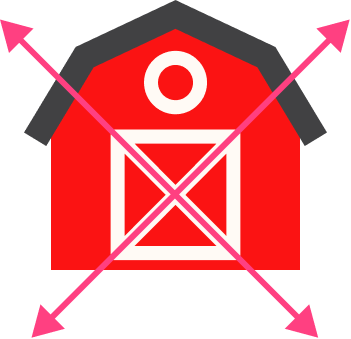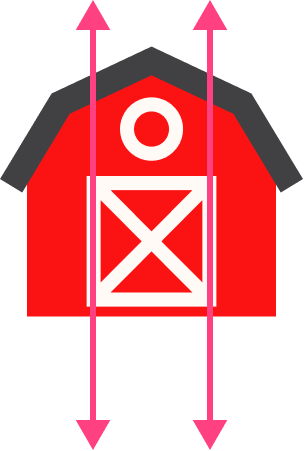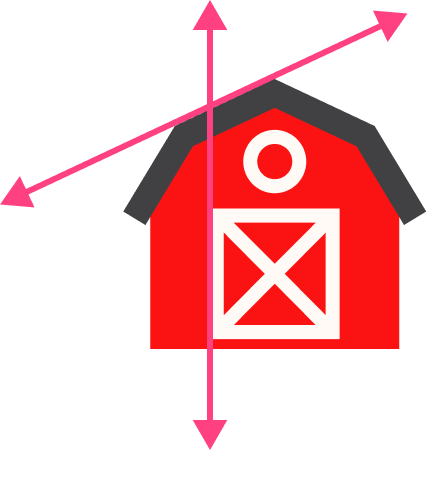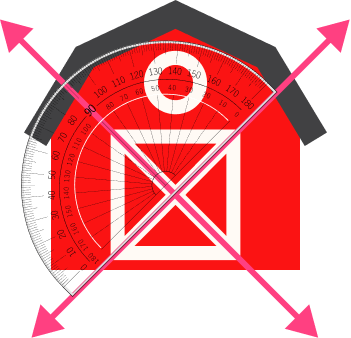Measuring with the protractor proves that there are four right angles (90^{\circ}) formed by the crossing lines, so these lines are perpendicular.

## Perpendicular lines FAQs

What is the perpendicular symbol?

The symbol used to represent perpendicular lines is ⊥.

What is the point of intersection?

This is where lines meet and form a point and an angle. For perpendicular lines, a 90 degree angle is formed.

How do students extend upon this knowledge in later grades?

The perpendicular relationship between lines or line segments is something students will continue to examine as they progress through middle and high school. When studying triangles, they will explore perpendicular bisectors.

When studying circles, they will explore the relationship between the tangent line and the radius of a circle. As they move through algebra they will calculate the slope and find the equation of perpendicular lines.

## Still stuck?

At Third Space Learning, we specialize in helping teachers and school leaders to provide personalized math support for more of their students through high-quality, online one-on-one math tutoring delivered by subject experts.

Each week, our tutors support thousands of students who are at risk of not meeting their grade-level expectations, and help accelerate their progress and boost their confidence.